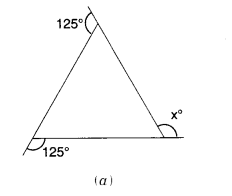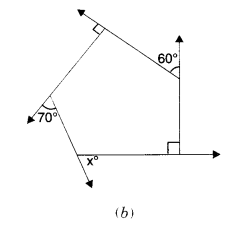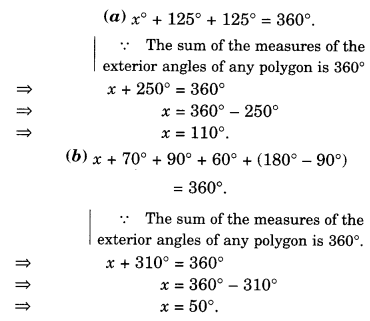# NCERT Solutions for Class 8 Maths Chapter 3 Understanding Quadrilaterals Ex 3.2

NCERT Solutions for Class 8 Maths Chapter 3 Understanding Quadrilaterals Ex 3.2 are part of NCERT Solutions for Class 8 Maths. Here we have given NCERT Solutions for Class 8 Maths Chapter 3 Understanding Quadrilaterals Ex 3.2.

 Board CBSE Textbook NCERT Class Class 8 Subject Maths Chapter Chapter 3 Chapter Name Understanding Quadrilaterals Exercise Ex 3.2 Number of Questions Solved 6 Category NCERT Solutions

## NCERT Solutions for Class 8 Maths Chapter 3 Understanding Quadrilaterals Ex 3.2

Question 1.
Find x in the following figures.Solution.Question 2.
Find the measure of each exterior angle of a regular polygon of
(i) 9 sides
(ii) 15 sides
Solution.
(i) 9 sides
Measure of each exterior angle=$$\frac { { 360 }^{ \circ } }{ 9 } ={ 40 }^{ \circ }$$

(ii) 15 slides
Measure of each exterior angle=$$\frac { { 360 }^{ \circ } }{ 15 } ={ 24 }^{ \circ }$$

Question 3.
How many sides does a regular polygon have if the measure of an exterior angle is 24°?
Solution.
Let the number of sides be n. Then, n(24°)=360°.
⇒ $$n=\frac { { 360 }^{ \circ } }{ 24 } =15$$
Hence, the number of sides is 15.

Question 4.
How many sides does a regular polygon have if each of its interior angles is 165°?
Solution.
∵ Each interior angle=165°
∴ Each exterior angle
= 180°-165°=15°
linear pair property
Let the number of sides be n. Then,
n(15°)=360°
$$n=\frac { { 360 }^{ \circ } }{ { 15 }^{ \circ } } =24$$
Hence, the number of sides is 24.

Question 5.
(a) Is it possible to have a regular polygon with a measure of each exterior angle at 22°?
(b) Can it be an interior angle of a regular polygon? Why?
Solution.
(a) No ; (since 22 is not a factor of 360).
(b) No ; (because each exterior angle is 180° – 22° = 158°, which is not a factor of 360°).

Question 6.
(a) What is the minimum interior angle possible for a regular polygon? Why?
(b) What is the maximum exterior angle possible for a regular polygon?
Solution.
(a) The equilateral triangle is a regular polygon of 3 sides has the minimum measure of an interior angle = 60°.
(b) By (a), we can see that the maximum exterior angle possible for a regular polygon is 180° – 60° = 120°.

We hope the NCERT Solutions for Class 8 Maths Chapter 3 Understanding Quadrilaterals Ex 3.2 help you. If you have any query regarding NCERT Solutions for Class 8 Maths Chapter 3 Understanding Quadrilaterals Ex 3.2, drop a comment below and we will get back to you at the earliest.